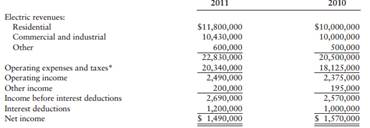### Create an Account

Home / Questions / Super Power Company reported the following statistics in its statements of income Requi...

# Super Power Company reported the following statistics in its statements of income Required a Calculate the operating ratio and comment on the results b Calculate the times interest earned and

Super Power Company reported the following statistics in its statements of income:Required

a. Calculate the operating ratio and comment on the results.

b. Calculate the times interest earned and comment on the results.

c. Perform a vertical common-size analysis of revenues, using total revenue as the base, and comment on the relative size of the component parts.

Apr 02 2020 View more View LessGet Solution